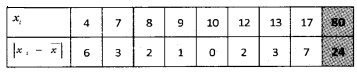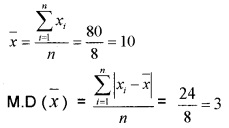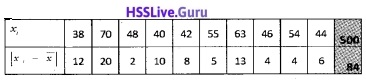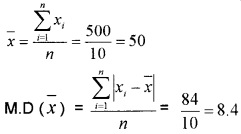# Plus One Maths Chapter Wise Questions and Answers Chapter 15 Statistics

Students can Download Chapter 15 Statistics Questions and Answers, Plus One Maths Chapter Wise Questions and Answers helps you to revise the complete Kerala State Syllabus and score more marks in your examinations.

## Kerala Plus One Maths Chapter Wise Questions and Answers Chapter 15 Statistics

### Plus One Maths Statistics Three Mark Questions and Answers

Question 1.
Find the mean deviation about the median for the following data: (3 score each)

1. 13, 17, 16, 14, 11, 13, 10, 16, 11, 18, 12, 17.
2. 36, 72, 46, 42, 60, 45, 53, 46, 51, 49.

1. Arrange the data in the ascending order we have;
10, 11, 11, 12, 13, 13, 14, 16, 16, 17, 17, 18
Here n = 12. So median is the average of 6th and 7th observations.
Therefore; Median, M =$$\frac{13+14}{2}$$ = 13.5Mean deviation = $$\frac{\sum_{i=1}^{n}\left|x_{i}-M\right|}{n}=\frac{28}{12}$$ = 2.33

2. Arrange the data in the ascending order we have; 36, 42, 45, 46, 46, 49, 51, 53, 60, 72
Here n = 10. So median is the average of 5th and 6th observations.
Therefore; Median, M = $$\frac{46+49}{2}$$ = 47.5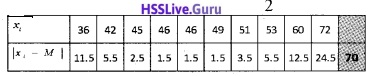Mean deviation = $$\frac{\sum_{i=1}^{n}\left|x_{i}-M\right|}{n}=\frac{70}{10}$$ = 7.Question 2.
The mean and standard deviation of marks obtained by 50 students of a class in three subjects, mathematics, Physics, and Chemistry are given below: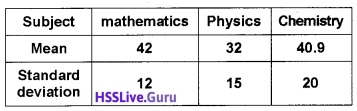Which of the three subject shows the highest variability in marks and which shows the lowest?
For Mathematics:
$$\bar{x}$$ = 42, σ = 12
∴ CV of Mathematics = $$\frac{12}{42}$$ × 100 = 28.57%
For Physics:
$$\bar{x}$$ = 32, σ = 15
∴ CV of Physics = $$\frac{15}{32}$$ × 100 = 46.88%
For Chemistry:
$$\bar{x}$$ = 40.9, σ = 20
∴ CV of Chemistry = $$\frac{20}{40.9}$$ × 100 = 48.9%
Thus Chemistry with highest CV shows highest variability and Mathematics with lowest CV shows lowest variability.

### Plus One Maths Statistics Four Mark Questions and Answers

Question 1.
Find the mean deviation about the mean for the following data: (4 score each)
1.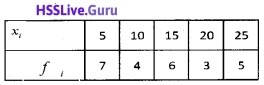2.1.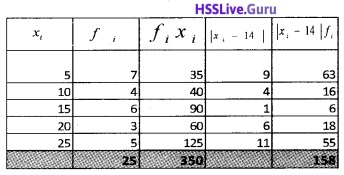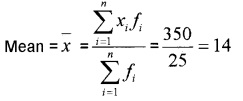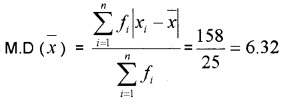2.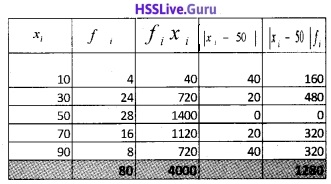Question 2.
Find the mean deviation about the median for the following data: (4 score each)
1.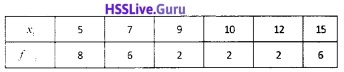2.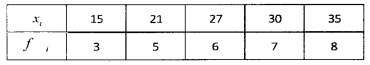1.$$\frac{\sum_{i=1}^{n} f_{i}}{2}=\frac{26}{2}$$ = 13
The c.f just greater than 13 is 14 and corresponding value of x is 7. Therefore; median, M = 7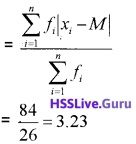2.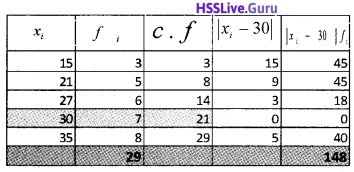$$\frac{\sum_{i=1}^{n} f_{i}}{2}=\frac{29}{2}$$ = 14.5
The c.f just greater than 14.5 is 21 and corresponding value of x is 30. Therefore; median, M = 30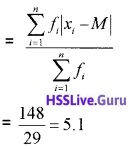Question 3.
Find the mean deviation about the mean for the following data: (4 score each)
1.2.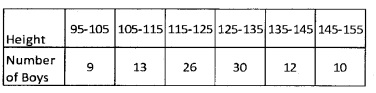1.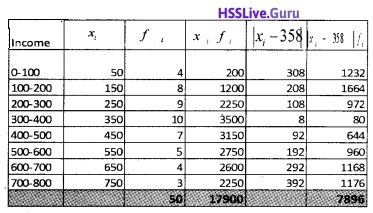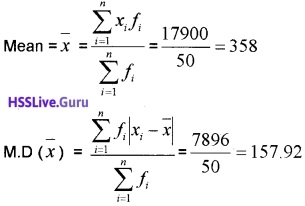2.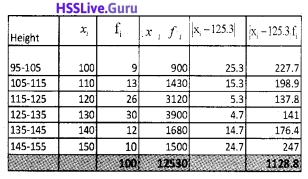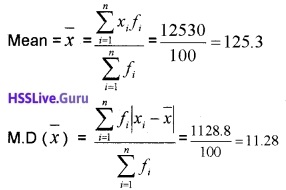Question 4.
Find the mean deviation about the median for the following data: (4 score each)
1.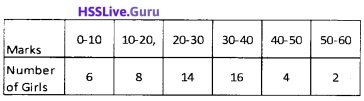2.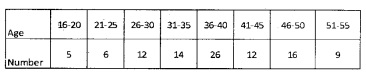1.Median class is the class in which the $$\left(\frac{N}{2}\right)^{th}$$ observation lies.
$$\frac{N}{2}=\frac{50}{2}$$ = 25
Median class = 20 – 30
M = Median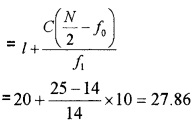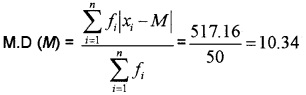2.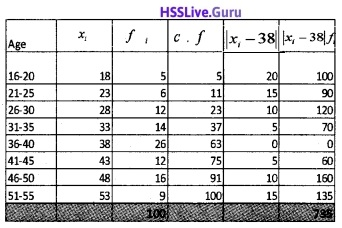Median class is the class in which the $$\left(\frac{N}{2}\right)^{th}$$ observation lies.
$$\frac{N}{2}=\frac{100}{2}$$ = 50
Median class = 35.5 – 40.5
M = Median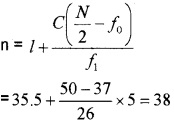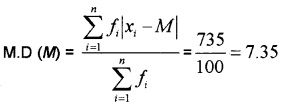Question 5.
Find the variance and standard deviation of 3, 4, 6, 5, 5, 3, 8, 1, 7, 5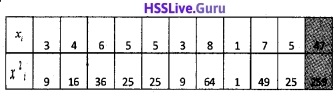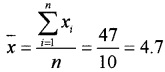$$\frac{259}{10}$$ – (4.7)2 = 25.9 – 22.09 = 3.8
Standard Deviation (σ) = $$\sqrt{\text {Variance}}$$ = $$\sqrt{3.8}$$ =1.95.Question 6.
Find the variance and standard deviation of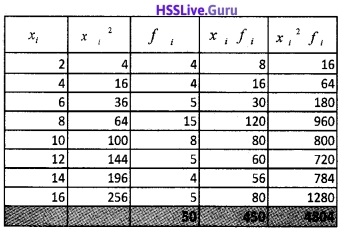Standard Deviation (σ) = $$\sqrt{\text {Variance}}$$
= $$\sqrt{15.08}$$ = 3.88.Question 7.
An analysis of monthly wages paid to workers in two firms A and B, belonging to the same industry, gives the following result.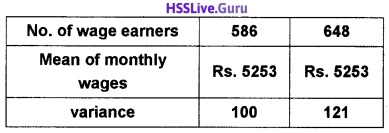1. Which firm A or B pays larger amount as monthly wages?
2. Which firm A or B, shows greater variability in individual wages?

1. Firm: A
Number of wages earners (n1) = 586
Number of wages earners ($$\bar{x}_{1}$$) = 5253
Total monthly wages = 5253 × 586 = 3078258
Firm: B
Number of wages earners (n1) = 648
Number of wages earners ($$\bar{x}_{1}$$) = 5253
Total monthly wages = 5253 × 648 = 3403944

2. Since both the firms have same mean of monthly wages, so the firm with greater variance will have more variability in individual wages. Thus firm B will have more variability in individual wages.Question 8.
The sum and sum of squares corresponding to length x (in cm) and weight y (in gm) of 50 plant products are given below: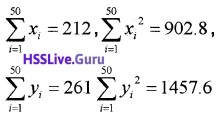which is more varying, the length or weight?
Here,Now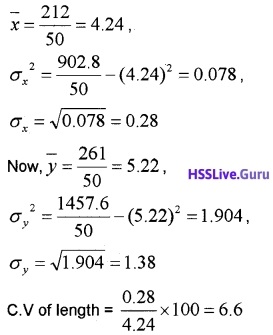C.V of weight = $$\frac{1.38}{5.22}$$ × 100 = 26.44
C.V of weight > C.V of length
Thus weight have more variability than length

### Plus One Maths Statistics Six Mark Questions and Answers

Question 1.
Find the variance and standard deviation of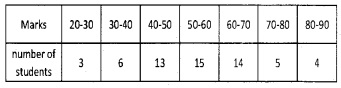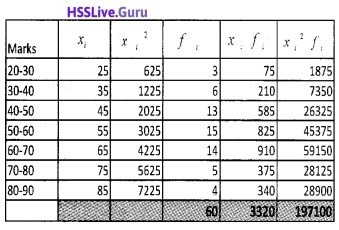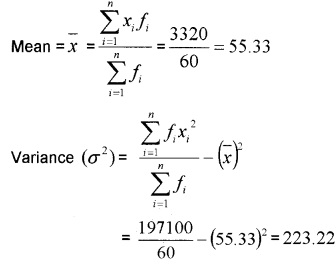Standard Deviation (a) = $$\sqrt{\text {Variance}}$$
= $$\sqrt{223.22}$$ = 14.94.

### Plus One Maths Statistics Practice Problems Questions and Answers

Question 1.
Find the mean deviation about the mean for the following data: (2 score each)

1. 4, 7, 8, 9, 10, 12, 13, 17.
2. 38, 70, 48, 40, 42, 55, 63, 46, 54, 44.